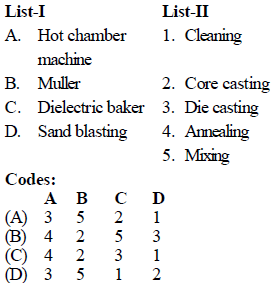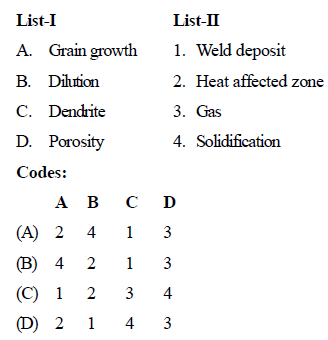Courses

# Test: Production Level - 3

## 25 Questions MCQ Test Mechanical Engineering SSC JE (Technical) | Test: Production Level - 3

Description
This mock test of Test: Production Level - 3 for Mechanical Engineering helps you for every Mechanical Engineering entrance exam. This contains 25 Multiple Choice Questions for Mechanical Engineering Test: Production Level - 3 (mcq) to study with solutions a complete question bank. The solved questions answers in this Test: Production Level - 3 quiz give you a good mix of easy questions and tough questions. Mechanical Engineering students definitely take this Test: Production Level - 3 exercise for a better result in the exam. You can find other Test: Production Level - 3 extra questions, long questions & short questions for Mechanical Engineering on EduRev as well by searching above.
QUESTION: 1

Solution:
QUESTION: 2

Solution:
QUESTION: 3

### Which of the following gating ratio represents pressurised gating systems ?

Solution:
QUESTION: 4

Arrange the following materials in order of their increasing die life :

Solution:
QUESTION: 5

Most commonly used materials cast by using hot-chamber die casting include

Solution:
QUESTION: 6

Match the List-I (Equipment) with List-II (Functions):Solution:
QUESTION: 7

Which of the following materials requires highest shrinkage allowance ?

Solution:
QUESTION: 8

Chills are used in casting moulds to

Solution:
QUESTION: 9

Chaplets are used in mould for

Solution:
QUESTION: 10

In shielded arc welding

Solution:
QUESTION: 11

Fluidity is greatly influenced by

Solution:
QUESTION: 12

The two parts of a sand casting mould are called

Solution:
QUESTION: 13

Which of the following are the most likely characteristics in centrifugal casting ?

Solution:
QUESTION: 14

Core prints are used in the casting

Solution:
QUESTION: 15

Match List-I with List-II and select the correct answer using the codes given below the lists :Solution:
QUESTION: 16

Consider the following stresses :
1. Tensile stress
2. Compressive stress
3. Shear stress
In forming processes, metals are subjected to

Solution:
QUESTION: 17

Warm metal working is applied extensively for

Solution:
QUESTION: 18

Thick-walled seamless tube is produced by

Solution:
QUESTION: 19

The process of cutting a flat sheet to the desired shape is known as

Solution:
QUESTION: 20

All of the following are squeezing operations, except

Solution:
QUESTION: 21

The size of a press is specified of

Solution:
QUESTION: 22

Blanking and drawing operations can be performed at one station of the press in the stroke of the ram in a

Solution:
QUESTION: 23

Which is not the application of forging ?

Solution:
QUESTION: 24

The process of putting a rod through several dies of decreasing diameter is known as

Solution:
QUESTION: 25

Dies for wire drawing are made of

Solution: